# Picturing Factors in Different Orders

Alignments to Content Standards: 5.OA.A

1. Find all the factor pairs for 30. For each factor pair, draw a picture that shows both of the factors as well as the product. For example, $3\times10=30$ and this picture shows 3 groups of 10 circles for a total of 30 circles: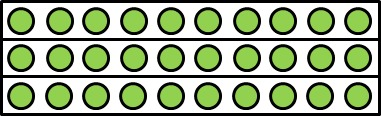Make sure you have a picture for both ways of ordering the factors in each pair. For example, make sure you have a picture that represents $10\times3=30$ as well as one that represents $3\times10=30$. You can use the same picture if you can explain how it shows the product written in the other order.

2. We can also write 30 as a product of three factors. For example, $$30=2\times(3\times5)$$ If we think of this as 2 groups with (3 groups of 5 circles in each group), then we can draw a picture that shows this: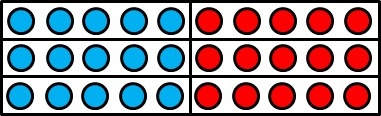We can change the order of these three factors as well: $$30 = 2\times(5\times3)$$ $$30 = 3\times(2\times5)$$ $$30 = 3\times(5\times2)$$ $$30 = 5\times(2\times3)$$ $$30 = 5\times(3\times2)$$ Draw a picture for each one that reflects the order of the factors.

## IM Commentary

The purpose of this task is to help students picture the multiplicative structure of the number 30 in different ways. Some of this structure is easier to communicate using grouping symbols, as it is easier to interpret $2\times(3\times5)$ than $2\times3\times5$ in terms of equal-sized groups even though they both mean "2 times 3 times 5." Because there are many different ways to draw the pictures called for in this task and it is the interpretation of the pictures that matters most, the task is very well suited for a whole-group discussion where students show and explain the pictures they have drawn to their classmates.

The pictures shown in the solution are increasingly more abstract as they go on. The first pictures in each part try to make the groups more explicit by using colors or divisions within the array; as the solution continues, the partitions of the array are described verbally. In this way, students can see that if they start with the same array, they can partition it in different ways to bring out different factors. If the teacher has 1/4-inch graph paper and colored pencils on hand, it will be much easier for students to draw the pictures called for in this task than if they are required to draw them free-hand.

The term "factor" has two different meanings: in the context of whole-number multiplication, a factor of a whole number is another whole number that divides it evenly (that is, with remainder 0). On the other hand, in the context of any explicitly defined multiplication problem

$$A\times B = C$$

we call $A$ and $B$ the factors and $C$ the product, even when $A$, $B$, and $C$ are not whole numbers. In fifth grade, students extend their understanding of multiplication to include factors that are not whole numbers, but this task invokes the first meaning, namely, whole-number factors. This task is a good precursor to 6.NS.B.4, where students are expected to find common factors and multiples, and thus need to be very comfortable with equations like

$$70 = 2\times5\times7$$

One of the best geometric representations of a number with three factors is as the volume of a rectangular prism, which is also important in 5th grade. See 5.MD,OA You Can Multiply Three Numbers in Any Order for a task that connects the different ways of multiplying the prime factors of 30 to the rectangular prism with dimensions 2, 3, and 5 units.

## Solution

1. All of the factor pairs of 30 include 1 and 30, 2 and 15, 3 and 10, and 5 and 6.

We have already seen $3\times10=30$ in the problem statement:To show $10\times3=30$, we could draw a very similar picture based on the same array that shows 10 groups of 3: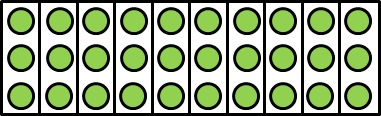For $5\times6=6\times5=30$, we can draw 5 groups of 6 or 6 groups of 5: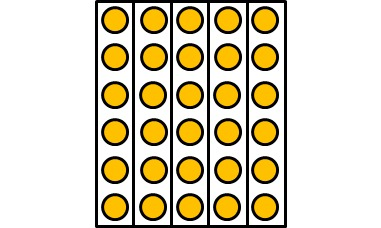A picture for $1\times30=30\times1=30$ is shown below:The picture can be thought of as 1 group of 30 circles or 30 groups with 1 circle in each group.

For $2\times15=15\times2=30$, we can draw the following picture: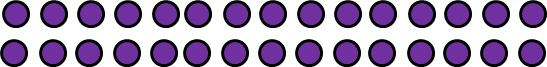Note that we can look at the same picture and see it as 2 groups of 15 (2 rows with 15 circles in each row) or 15 groups of 2 (15 columns with 2 circles in each column.) We could have done the same thing with the other pictures, but we wanted to show the groups more explicitly.

2. The task statement shows a picture for $30 = 2 \times (3 \times 5)$ because there are two groups (a blue group and a red group) with 3 rows and 5 circles in each row:We can also draw it like this: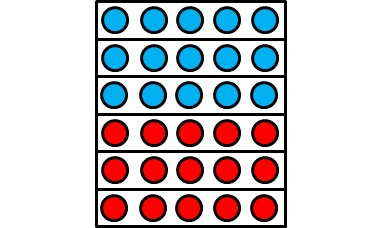• Here is a picture for $30 = 2\times(5\times3)$ which is built from an array like the one we drew for $2 \times (3 \times 5)$ except the groups are different because we have 2 groups with 5 columns that each contain 3 circles:We can also draw it like this:There are many other ways to represent this product.

• This is the picture for $3\times10$ with a vertical line drawn down the middle to show 3 rows with 2 groups of 5 circles in each row: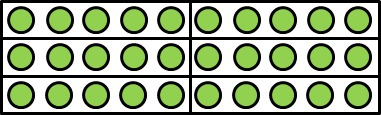which shows that $30=3\times(2\times5)$. There are many other ways to represent this product.

• Similarly, we can take the picture for $3\times10$ and subdivide it into 5 pieces, and then see that the 3 rows each contain 5 groups with 2 circles in each, which shows that $30=3\times(5\times2)$:There are many other ways to represent this product.

• This picture is the picture for $5\times6$ with a horizontal line drawn across the middle to show $30=5\times(2\times3)$. We can see 5 columns with 2 groups of 3 circles in each column:Note that this is the same as the second picture that we used to show $30 = 2\times(5\times3)$ but with different coloring. This shows that it is the interpretation of a picture that is most important for establishing the mathematical meaning.

There are many other ways to represent this product.

• We can take the picture we used to show $15\times2$ and break it into 5 groups with 3 columns and 2 circles in each column for $30=5\times(3\times2)$.There are many other ways to represent this product.